Main Content

# Expected Shortfall (ES) Backtesting Workflow Using Simulation

This example shows an expected shortfall (ES) backtesting workflow using the `esbacktestbysim` object. The tests supported in the `esbacktestbysim` object require as inputs not only the test data (`Portfolio`, `VaR`, and `ES` data), but also the distribution information of the model being tested.

The `esbacktestbysim` class supports five tests -- conditional, unconditional, quantile, which are based on Acerbi-Szekely (2014) and minBiasAbsolute and minBiasRelative, which are based on Acerbi-Szekely (2017 ans 2019). These tests use the distributional assumptions to simulate return scenarios, assuming the distributional assumptions are correct (null hypothesis). The simulated scenarios find the distribution of typical values for the test statistics and the significance of the tests. `esbacktestbysim` supports normal and t location-scale distributions (with a fixed number of degrees of freedom throughout the test window).

### Step 1. Load the ES backtesting data.

Use the `ESBacktestBySimData.mat` file to load the data into the workspace. This example works with the `Returns` numeric array. This array represents the equity returns. The corresponding VaR data and VaR confidence levels are in `VaR` and `VaRLevel`. The expected shortfall data is contained in `ES`.

`load ESBacktestBySimData`

### Step 2. Generate an ES backtesting plot.

Use the `plot` function to visualize the ES backtesting data. This type of visualization is a common first step when performing an ES backtesting analysis. This plot displays the returns data against the VaR and ES data.

```VaRInd = 2; figure; plot(Dates,Returns,Dates,-VaR(:,VaRInd),Dates,-ES(:,VaRInd)) legend('Returns','VaR','ES') title(['Test Data, ' num2str(VaRLevel(VaRInd)*100) '% Confidence']) grid on```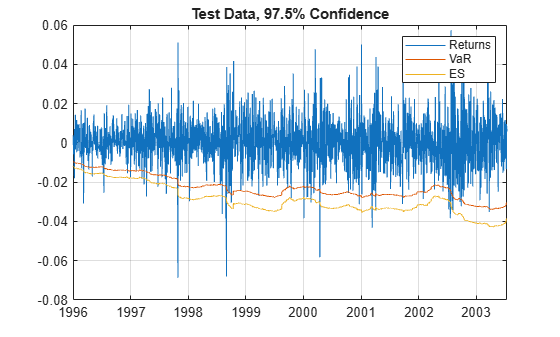### Step 3. Create an `esbacktestbysim` object.

Create an `esbacktestbysim` object using `esbacktestbysim`. The `Distribution` information is used to simulate returns to estimate the significance of the tests. The simulation to estimate the significance is run by default when you create the `esbacktestbysim` object. Therefore, the test results are available when you create the object. You can set the optional name-value pair input argument `'Simulate'` to `false` to avoid the simulation, in which case you can use the `simulate` function before querying for test results.

```rng('default'); % for reproducibility IDs = ["t(dof) 95%","t(dof) 97.5%","t(dof) 99%"]; IDs = strrep(IDs,"dof",num2str(DoF)); ebts = esbacktestbysim(Returns,VaR,ES,Distribution,... 'DegreesOfFreedom',DoF,... 'Location',Mu,... 'Scale',Sigma,... 'PortfolioID',"S&P",... 'VaRID',IDs,... 'VaRLevel',VaRLevel); disp(ebts)```
``` esbacktestbysim with properties: PortfolioData: [1966x1 double] VaRData: [1966x3 double] ESData: [1966x3 double] Distribution: [1x1 struct] PortfolioID: "S&P" VaRID: ["t(10) 95%" "t(10) 97.5%" "t(10) 99%"] VaRLevel: [0.9500 0.9750 0.9900] ```
`disp(ebts.Distribution) % distribution information stored in the 'Distribution' property`
``` Name: "t" DegreesOfFreedom: 10 Location: 0 Scale: [1966x1 double] ```

### Step 4. Generate the ES summary report.

The ES summary report provides information about the severity of the violations, that is, how large the loss is compared to the VaR on days when the VaR was violated. The `ObservedSeverity` (or observed average severity ratio) column is the ratio of loss to VaR over days when the VaR is violated. The `ExpectedSeverity` (or expected average severity ratio) column shows the average of the ratio of ES to VaR on the days when the VaR is violated.

```S = summary(ebts); disp(S)```
``` PortfolioID VaRID VaRLevel ObservedLevel ExpectedSeverity ObservedSeverity Observations Failures Expected Ratio Missing ___________ _____________ ________ _____________ ________________ ________________ ____________ ________ ________ ______ _______ "S&P" "t(10) 95%" 0.95 0.94812 1.3288 1.4515 1966 102 98.3 1.0376 0 "S&P" "t(10) 97.5%" 0.975 0.97202 1.2652 1.4134 1966 55 49.15 1.119 0 "S&P" "t(10) 99%" 0.99 0.98627 1.2169 1.3947 1966 27 19.66 1.3733 0 ```

### Step 5. Run a report for all tests.

Run all tests and generate a report on only the accept or reject results.

```t = runtests(ebts); disp(t)```
``` PortfolioID VaRID VaRLevel Conditional Unconditional Quantile MinBiasAbsolute MinBiasRelative ___________ _____________ ________ ___________ _____________ ________ _______________ _______________ "S&P" "t(10) 95%" 0.95 reject accept reject accept reject "S&P" "t(10) 97.5%" 0.975 reject reject reject reject reject "S&P" "t(10) 99%" 0.99 reject reject reject reject reject ```

### Step 6. Run the conditional test.

Run the individual test for the conditional test (also known as the first Acerbi-Szekely test). The second output (`s`) contains simulated test statistic values, assuming the distributional assumptions are correct. Each row of the `s` output matches the `VaRID` in the corresponding row of the `t` output. Use these simulated statistics to determine the significance of the tests.

```[t,s] = conditional(ebts); disp(t)```
``` PortfolioID VaRID VaRLevel Conditional ConditionalOnly PValue TestStatistic CriticalValue VaRTest VaRTestResult VaRTestPValue Observations Scenarios TestLevel ___________ _____________ ________ ___________ _______________ ______ _____________ _____________ _______ _____________ _____________ ____________ _________ _________ "S&P" "t(10) 95%" 0.95 reject reject 0 -0.092302 -0.043941 "pof" accept 0.70347 1966 1000 0.95 "S&P" "t(10) 97.5%" 0.975 reject reject 0.001 -0.11714 -0.052575 "pof" accept 0.40682 1966 1000 0.95 "S&P" "t(10) 99%" 0.99 reject reject 0.003 -0.14608 -0.085433 "pof" accept 0.11536 1966 1000 0.95 ```
`whos s`
``` Name Size Bytes Class Attributes s 3x1000 24000 double ```

### Step 7. Visualize the significance of the conditional test.

Visualize the significance of the conditional test using histograms to show the distribution of typical values (simulation results). In the histograms, the asterisk shows the value of the test statistic observed for the actual returns. This is a visualization of the standalone conditional test. The final conditional test result also depends on a preliminary VaR backtest, as shown in the conditional test output.

```NumVaRs = height(t); figure; for VaRInd = 1:NumVaRs subplot(NumVaRs,1,VaRInd) histogram(s(VaRInd,:)); hold on; plot(t.TestStatistic(VaRInd),0,'*'); hold off; Title = sprintf('Conditional: %s, p-value: %4.3f',t.VaRID(VaRInd),t.PValue(VaRInd)); title(Title) end```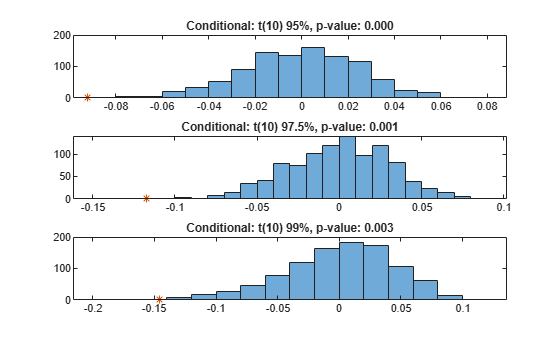### Step 8. Run the unconditional test.

Run the individual test for the unconditional test (also known as the second Acerbi-Szekely test).

```[t,s] = unconditional(ebts); disp(t)```
``` PortfolioID VaRID VaRLevel Unconditional PValue TestStatistic CriticalValue Observations Scenarios TestLevel ___________ _____________ ________ _____________ ______ _____________ _____________ ____________ _________ _________ "S&P" "t(10) 95%" 0.95 accept 0.093 -0.13342 -0.16252 1966 1000 0.95 "S&P" "t(10) 97.5%" 0.975 reject 0.031 -0.25011 -0.2268 1966 1000 0.95 "S&P" "t(10) 99%" 0.99 reject 0.008 -0.57396 -0.38264 1966 1000 0.95 ```

### Step 9. Visualize the significance of the unconditional test.

Visualize the significance of the unconditional test using histograms to show the distribution of typical values (simulation results). In the histograms, the asterisk shows the value of the test statistic observed for the actual returns.

```NumVaRs = height(t); figure; for VaRInd = 1:NumVaRs subplot(NumVaRs,1,VaRInd) histogram(s(VaRInd,:)); hold on; plot(t.TestStatistic(VaRInd),0,'*'); hold off; Title = sprintf('Unconditional: %s, p-value: %4.3f',t.VaRID(VaRInd),t.PValue(VaRInd)); title(Title) end```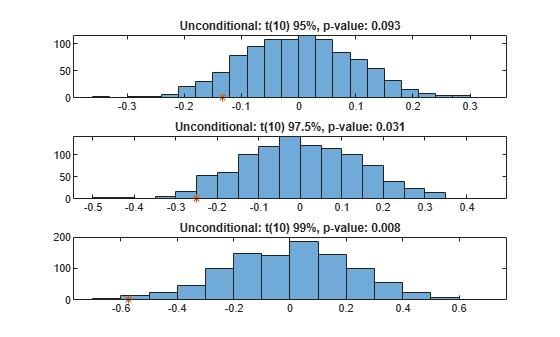### Step 10. Run the quantile test.

Run the individual test for the quantile test (also known as the third Acerbi-Szekely test).

```[t,s] = quantile(ebts); disp(t)```
``` PortfolioID VaRID VaRLevel Quantile PValue TestStatistic CriticalValue Observations Scenarios TestLevel ___________ _____________ ________ ________ ______ _____________ _____________ ____________ _________ _________ "S&P" "t(10) 95%" 0.95 reject 0.002 -0.10602 -0.055798 1966 1000 0.95 "S&P" "t(10) 97.5%" 0.975 reject 0 -0.15697 -0.073513 1966 1000 0.95 "S&P" "t(10) 99%" 0.99 reject 0 -0.26561 -0.10117 1966 1000 0.95 ```

### Step 11. Visualize the significance of the quantile test.

Visualize the significance of the quantile test using histograms to show the distribution of typical values (simulation results). In the histograms, the asterisk shows the value of the test statistic observed for the actual returns.

```NumVaRs = height(t); figure; for VaRInd = 1:NumVaRs subplot(NumVaRs,1,VaRInd) histogram(s(VaRInd,:)); hold on; plot(t.TestStatistic(VaRInd),0,'*'); hold off; Title = sprintf('Quantile: %s, p-value: %4.3f',t.VaRID(VaRInd),t.PValue(VaRInd)); title(Title) end```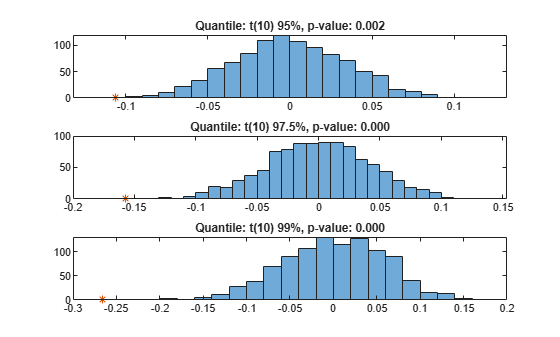### Step 10. Run the minBiasAbsolute test.

Run the individual test for the minBiasAbsolute test.

```[t,s] = minBiasAbsolute(ebts); disp(t)```
``` PortfolioID VaRID VaRLevel MinBiasAbsolute PValue TestStatistic CriticalValue Observations Scenarios TestLevel ___________ _____________ ________ _______________ ______ _____________ _____________ ____________ _________ _________ "S&P" "t(10) 95%" 0.95 accept 0.062 -0.0014247 -0.0015578 1966 1000 0.95 "S&P" "t(10) 97.5%" 0.975 reject 0.029 -0.0026674 -0.0023251 1966 1000 0.95 "S&P" "t(10) 99%" 0.99 reject 0.005 -0.0060982 -0.0039004 1966 1000 0.95 ```

### Step 11. Visualize the significance of the minBiasAbsolute test.

Visualize the significance of the minBiasAbsolute test using histograms to show the distribution of typical values (simulation results). In the histograms, the asterisk shows the value of the test statistic observed for the actual returns.

```NumVaRs = height(t); figure; for VaRInd = 1:NumVaRs subplot(NumVaRs,1,VaRInd) histogram(s(VaRInd,:)); hold on; plot(t.TestStatistic(VaRInd),0,'*'); hold off; Title = sprintf('minBiasAbsolute: %s, p-value: %4.3f',t.VaRID(VaRInd),t.PValue(VaRInd)); title(Title) end```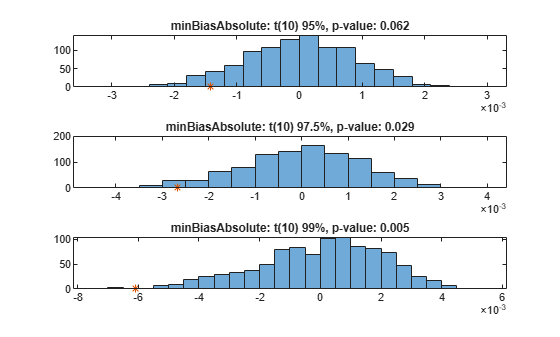### Step 10. Run the minBiasRelative test.

Run the individual test for the minBiasRelative test.

```[t,s] = minBiasRelative(ebts); disp(t)```
``` PortfolioID VaRID VaRLevel MinBiasRelative PValue TestStatistic CriticalValue Observations Scenarios TestLevel ___________ _____________ ________ _______________ ______ _____________ _____________ ____________ _________ _________ "S&P" "t(10) 95%" 0.95 reject 0.003 -0.10509 -0.056072 1966 1000 0.95 "S&P" "t(10) 97.5%" 0.975 reject 0 -0.15603 -0.073324 1966 1000 0.95 "S&P" "t(10) 99%" 0.99 reject 0 -0.26716 -0.104 1966 1000 0.95 ```

### Step 11. Visualize the significance of the minBiasAbsolute test.

Visualize the significance of the minBiasRelative test using histograms to show the distribution of typical values (simulation results). In the histograms, the asterisk shows the value of the test statistic observed for the actual returns.

```NumVaRs = height(t); figure; for VaRInd = 1:NumVaRs subplot(NumVaRs,1,VaRInd) histogram(s(VaRInd,:)); hold on; plot(t.TestStatistic(VaRInd),0,'*'); hold off; Title = sprintf('minBiasRelative: %s, p-value: %4.3f',t.VaRID(VaRInd),t.PValue(VaRInd)); title(Title) end```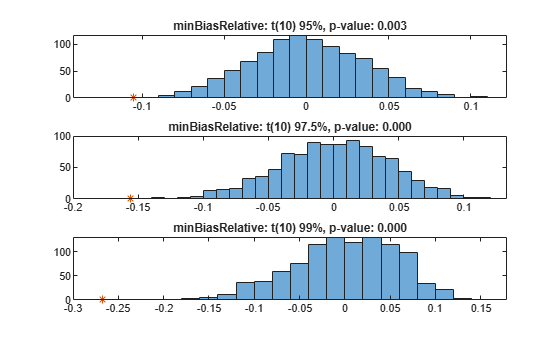### Step 12. Run a new simulation to estimate the significance of the tests.

Run the simulation again using 5000 scenarios to generate a new set of test results. If the initial test results for one of the tests are borderline, using a larger simulation can help clarify the test results.

```ebts = simulate(ebts,'NumScenarios',5000); t = unconditional(ebts); % new results for unconditional test disp(t)```
``` PortfolioID VaRID VaRLevel Unconditional PValue TestStatistic CriticalValue Observations Scenarios TestLevel ___________ _____________ ________ _____________ ______ _____________ _____________ ____________ _________ _________ "S&P" "t(10) 95%" 0.95 accept 0.0984 -0.13342 -0.17216 1966 5000 0.95 "S&P" "t(10) 97.5%" 0.975 reject 0.0456 -0.25011 -0.24251 1966 5000 0.95 "S&P" "t(10) 99%" 0.99 reject 0.0104 -0.57396 -0.40089 1966 5000 0.95 ```

## Support

#### Effective Model Risk Management with MATLAB

Download white paper